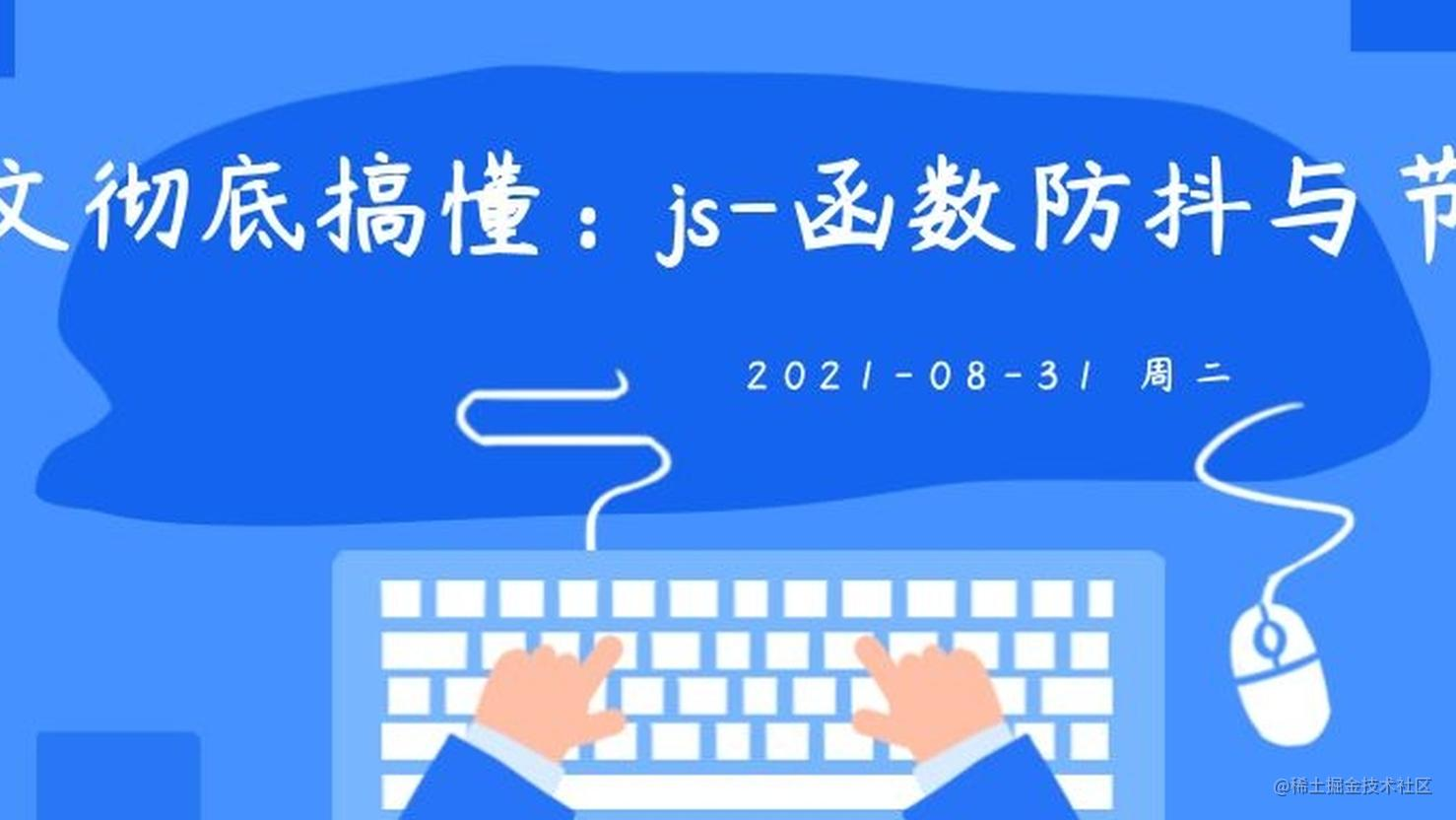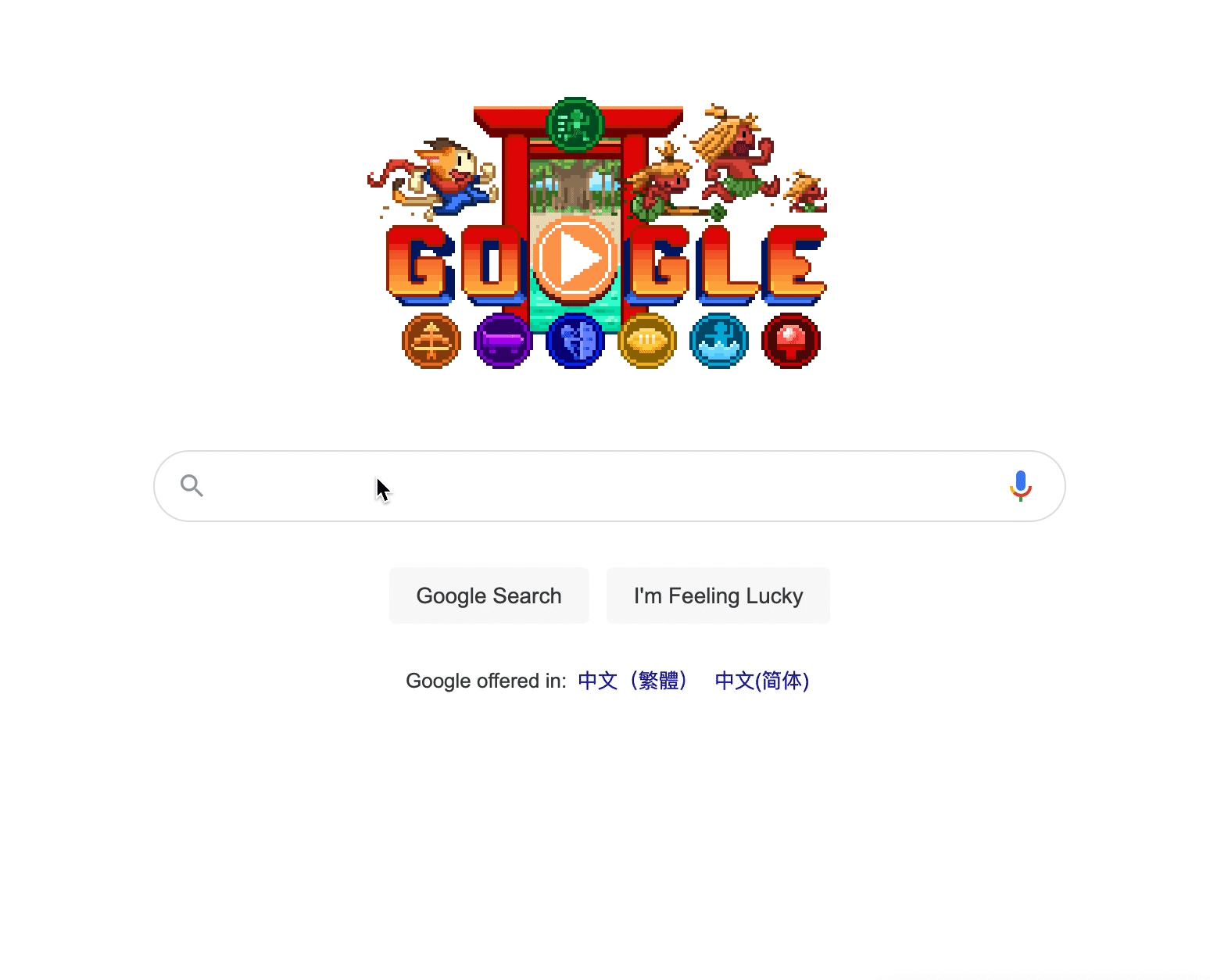# 一文彻底搞懂：js函数防抖与节流### 防抖``````<!DOCTYPE html>
<html lang="en">
<meta charset="UTF-8">
<meta name="viewport" content="width=device-width, initial-scale=1.0">
<title>函数防抖</title>
<body>
<input id='input'/>
<script>
const input = document.getElementById('input')
let timerId = null
input.oninput = function (event) {
console.log(event.srcElement.value)
}
</script>
</body>
</html>

``````let timerId = null
input.oninput = function (event) {
if (timerId) {
clearTimeout(timerId)
}
timerId = setTimeout(() => {
console.log(event.srcElement.value)
}, 1000);
}

``````function debounce(fn, delay) {
let timerId = null
let self = this
return function() {
if (timerId) {
clearTimeout(timerId)
}
let args = arguments
timerId = setTimeout(() => {
fn.apply(self, args)
}, time);
}
}

``````function test(event) {
console.log(event)
}
input.oninput = debounce(test, 1000)

### 节流

``````const input = document.getElementById('root')

function debounce(fn, time) {
let timerId = null
let self = this
return function() {
if (timerId) {
clearTimeout(timerId)
}
let args = arguments
timerId = setTimeout(() => {
fn.apply(self, args)
}, time);
}
}

function reSetSize() {
let width = window.innerWidth
let height = window.innerHeight
input.style.width = width/2 + 'px'
input.style.height = height/2 + 'px'
}
reSetSize()
window.onresize = debounce(reSetSize, 1000);

``````function throttle(fn, time) {
let flag = true
let timerId = null
let self = this
return function() {
if (!flag) {
return
}
flag = false
if (timerId) {
clearTimeout(timerId)
}
let args = arguments
timerId = setTimeout(() => {
flag = true
fn.apply(self, args)
}, time);
}
}

``````window.onresize = debounce(reSetSize, 1000);

### 总结

• `防抖` 当某一行为停止之后过n时长再执行响应的操作。
• `节流` 每个n时长就执行一次函数。